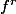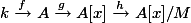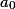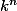# Martin Orr's Blog

Maths > Algebraic geometry > Nullstellenstatz

## Proof of the Nullstellensatz

Posted by Martin Orr on Friday, 19 June 2009 at 11:49

Hilbert's Nullstellensatz is an algebraic result fundamental to algebraic geometry. There are many different proofs of the Nullstellensatz. In this post I will consider the proof given in this year's Part III Commutative Algebra course, and in particular one section of the proof that seems to contain lots of magic. When I was revising for the exams, I realised that part of the mystery came from the fact that the particular theorem proved in Commutative Algebra does not require an algebraically closed field, unlike the standard statement of Hilbert's Nullstellensatz.

Thanks are due to Lloyd West for starting me thinking about this, and to Jon Nelson for giving me the courage to believe that it might be true and for supplying the proof of Lemma 4.

The standard statement of Hilbert's Nullstellensatz is:

Theorem 1. Let k be an algebraically closed field, let I be an ideal in `` and let f be a polynomial vanishing at all points of V(I). Then `` is in I for some integer r.

The theorem which was called the Nullstellensatz and proved in Commutative Algebra was:

Theorem 2. Let k be an algebraically closed field and A a finitely-generated k-algebra. Then Jac(A) = N(A) (where Jac(A) = intersection of all maximal ideals, N(A) = intersection of all prime ideals).

Getting from this statement to Theorem 1 is left as an exercise to the reader. Theorem 1 very definitely requires k to be algebraically closed, but it turns out that Theorem 2 remains true without this condition.

In proving Theorem 2, we used the following lemma which I shall assume:

Lemma 3. Let k be a field and A a finitely-generated k-algebra which is itself a field. Then A is an algebraic extension of k.

It is obvious that N(A) is contained in Jac(A). To go the other way, take a in Jac(A) and suppose that a is not in N(A). Then 1 - ax is not a unit in A[x], so there is a maximal ideal M of A[x] containing 1 - ax.

Now consider the following sequence of k-algebra homomorphisms:

``

This makes A[x]/M into a finitely-generated k-algebra, and it is a field because M is maximal. So by Lemma 3, A[x]/M is an algebraic extension of k.

Now we want to show that A/ker(hg) = Im(hg) is a field, so that ker(hg) is a maximal ideal in A. But hg(a)h(x) = 1 in A[x]/M, so a is not in ker(hg), contradicting a in Jac(A).

If we assume that k is algebraically closed, then A[x]/M being an algebraic extension of k tells us that A[x]/M is isomorphic to k, and hgf is an isomorphism. It follows that hg is a surjection and Im(hg) = A[x]/M is a field.

But even if k is not algebraically closed, so that hg is not a surjection, it turns out that Im(hg) is still a field and this proof works. We just need the following lemma (with L = A[x]/M, R = Im(hg), K = k):

Lemma 4. Let L/K be an algebraic field extension and R a ring such that ``. Then R is a field.

Proof. Given u in R (not 0): since L/K is algebraic, there is a polynomial `` over K with u as a root, and `` non-zero.

Then `` is in R.

And `` is invertible in K, so in R.

End of proof.

Geometrically, Theorem 2 tells us that when doing algebraic geometry over a field k it is enough to consider a space as being made up of its closed points (corresponding to maximal ideals) rather than requiring non-closed points (prime ideals). I was slightly surprised to discover that this is true even when k is not algebraically closed - this means that non-closed points only become essential when we consider schemes not defined over a field at all.

Instead, the need for algebraic closure comes in the step from Theorem 2 to Theorem 1. Geometrically, we get that over an algebraically closed field the closed points of affine n-space are simply the elements of ``. Over a non-algebraically closed field, there are additional closed points.